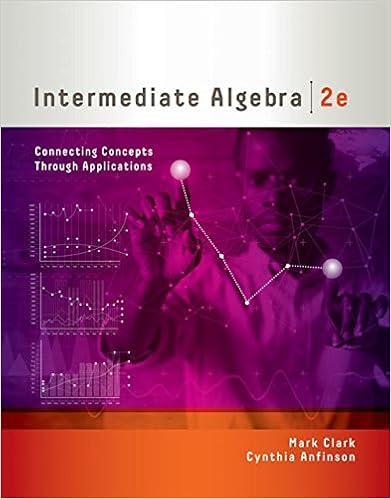# Quiz 3 Questions and Answer Key - Stat-312 Quiz#03 Name Key...

• Test Prep
• 2
• 100% (3) 3 out of 3 people found this document helpful

This preview shows page 1 - 2 out of 2 pages.

##### We have textbook solutions for you!
The document you are viewing contains questions related to this textbook.The document you are viewing contains questions related to this textbook.
Chapter 10 / Exercise 14
Intermediate Algebra: Connecting Concepts through Applications
ClarkExpert Verified
Stat-312: Quiz #03 Name: Key 1. A vending machine accepts nickels, dimes, and quarters, and rejects any objects that it does not recognize as one of these coins. Unfortunately, the machine also randomly rejects valid coins. The probability distribution for the number of times the machine rejects a valid coin is given in the table. k P(X=k) (a) What is the chance that the machine will reject a valid coin more than three times before the coin is accepted? (b) Calculate the mean and standard deviation for the distribution. (c) Draw the distribution in the space below. Draw the locations of the mean and standard deviation of the distribution in the distribution drawing. 2. On a multiple choice exam, there are five questions, and each question has four choices (only one of which is correct). Suppose that a really bad student comes to class without doing the homework or reading the text;
##### We have textbook solutions for you!
The document you are viewing contains questions related to this textbook.The document you are viewing contains questions related to this textbook.
Chapter 10 / Exercise 14
Intermediate Algebra: Connecting Concepts through Applications
ClarkExpert Verified
•••